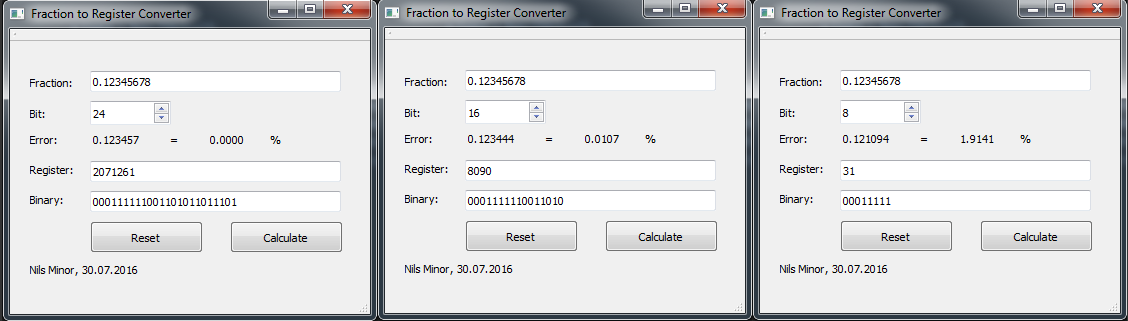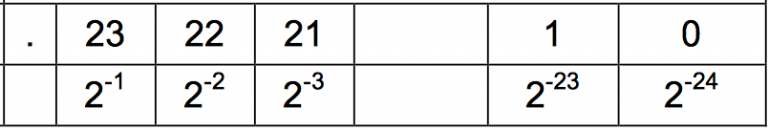Last year I wrote a tiny Qt application to convert a floating-point number to a register value. The picture below shows how to use the software. Simply fill out the “Fraction” field and press calculate. Default, the value will be build with 24Bit accuracy. You can see how the error increases, when the bit count is reduced. In the example the accuracy is reduced from 24 Bit, error below 0.00%, to 8 Bit accuracy with an error of 1.9%.The error appears to the reduced steps of the fraction number. You can see in the picture on the right, how a fraction number is build. 2^-1 = 0.5, 2^-2 = 0.25, 2^-3 = 0.125 an so on.The more bits used, so much better is the resolution and the error is reduced. Since I used this tool only for a micro controller based application, I want to share the code. You can find it on my Github page here.